# Test: Fluid Mechanics- 1

## 25 Questions MCQ Test Mechanical Engineering SSC JE (Technical) | Test: Fluid Mechanics- 1

Description
Attempt Test: Fluid Mechanics- 1 | 25 questions in 50 minutes | Mock test for Mechanical Engineering preparation | Free important questions MCQ to study Mechanical Engineering SSC JE (Technical) for Mechanical Engineering Exam | Download free PDF with solutions
QUESTION: 1

Solution:
QUESTION: 2

Solution:
QUESTION: 3

### Poise is a unit of –

Solution:
QUESTION: 4

With increase in temperature the viscosity of gases and liquids respectively : -

Solution:
QUESTION: 5

Below is shown a graph.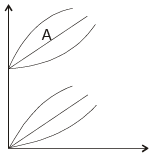The line marked 'A' represents

Solution:
QUESTION: 6

If P = Pressure r = density, then compressibility can be defined as,

Solution:
QUESTION: 7

A cold chisel is made of

Solution:
QUESTION: 8

Bourdon gauge is used to measure

Solution:
QUESTION: 9

pressure at a place was measured to be 1000 mm of mercury. The pressure in terms of height of water will be :-

Solution:
QUESTION: 10

In the shown diagram velocity V will be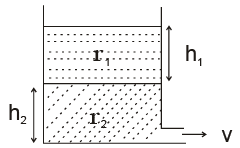Solution:
QUESTION: 11

A square plate of side 10 cm is immersed vertically in water with its top edge 5 cm below water surface. The total pressure force on the surface will be

Solution:
QUESTION: 12

If the plate in above question is moved down deeper into water the center of pressure will

Solution:
QUESTION: 13

A completely submerged body will be in stable equilibrium if :

B → Center of Buoyancy
G → Center of gravity
M → Metacenter..

Solution:
QUESTION: 14

A body of density 600 kg/m3 floats in a liquid of density 1000 kg/m3. The percentage of volume immersed in water is

Solution:
QUESTION: 15

Atmospheric pressure is equal to

Solution:
QUESTION: 16

Time period of transverse oscillation of a floating body is given as

KG → least radins of gyration.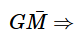Metacentric height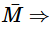Metacentric radius

Solution:
QUESTION: 17

Langrangian method of fluid study is also:-

Solution:
QUESTION: 18

For steady flow which of the following lines becomes identical
1. streamline
2. streak line
3. path line
4. Time line

Solution:
QUESTION: 19

The velocity at a point is given as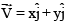The equation of stream line is

Solution:
QUESTION: 20

When a fluid flows in a curved path without any external force the fluid motion is called

Solution:
QUESTION: 21

The speed factor (speed ratio) of a Pelton turbine for its efficient operation lies in the range

Solution:
QUESTION: 22

A liquid of specific gravity 0.7 flows with a velocity of 5 m/s. Its velocity head is near to.

Solution:
QUESTION: 23

Bernoulis equation refers to conservation of –

Solution:
QUESTION: 24

Which of the following can be used to measure velocity in open channel flow.

Solution:
QUESTION: 25

Spyghmanometer is a device used to measure

Solution:Use Code STAYHOME200 and get INR 200 additional OFF Use Coupon Code